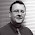## Friday, 3 April 2009

### Excel Tip: Using VLOOKUP

Second only to pivot tables, the VLOOKUP formula is the one of the features of Excel that I am most often asked about. It is also one of the most useful.

Simply put, this formula allows you to look up an item from a list.

Its format is as follows:

=VLOOKUP(lookup value,range to lookup,column to return,TRUE or FALSE)

lookup value: This is the value you wish to search the first column of your table for (this can be the actual value, but it can be very powerful to refer to a cell containing the value).

range to lookup: This is the range (either named, or referred to directly) that contains the lookup table. It is useful to refer to whole columns as this allows your list to grow.

column to return: This is given as a number, where the first column (the one being searched) is 1.

TRUE or FALSE: This is a rather odd one. If you enter 'TRUE' or omit this argument, Excel will go to the closest value if the actual value being looked up is not there. This requires the first column to be sorted in ascending order. I can think of very few applications for this other than some form of conversion table, but as conversion tables are normally to help approximate a formula, there is not a lot of call for them in Excel where you can easily calculate the actual formula itself. For most financial applications you will need to enter 'FALSE' here as this will return an #NA error if it cannot find the lookup value.

The VLOOKUP formula is very useful for adding additional data to raw imported data in Excel. For one application, see my article on automating management accounts.

1.The TRUE argument is very useful when you want to select a value in ranges - so if you have financial periods that are 4 weeks long rather than months, you can have a table with the first day of the month and the period number, and use VLOOKUP to fetermine the period.

2.In response to Anonymous:

That's a great use for VLOOKUP with the TRUE argument. Thanks for the comment.

3.My work demands the use of Vlookup function and now I can use it easily. Thanks for simplified information.

4.CCNA Training: Thanks for the positive comments. Pleased you found it useful.

There is a free video on VLOOKUP in a more recent post.